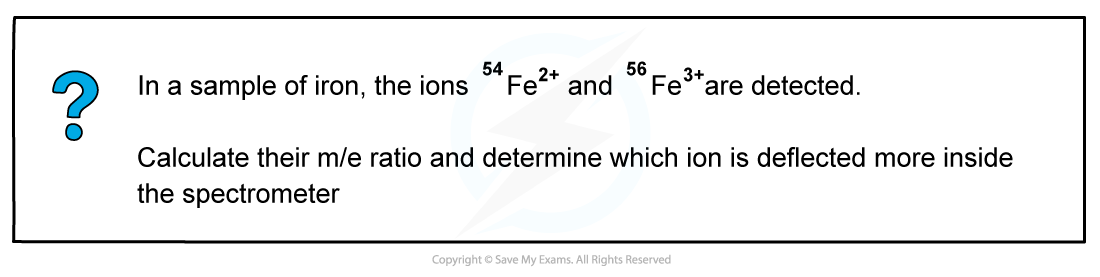# CIE A Level Chemistry复习笔记4.1.2 Mass Spectrometry

### Interpreting Mass Spectra

• Mass spectroscopy is an analytical technique used to identify unknown compounds
• The molecules in the small sample are bombarded with high energy electrons which can cause the molecule to lose an electron
• This results in the formation of a positively charged molecular ion with one unpaired electron
• One of the electrons in the pair has been removed by the beam of electrons• The molecular ion can further fragment to form new ions, molecules, and radicalsFragmentation of a molecule in mass spectroscopy

• These fragmentation ions are accelerated by an electric field
• Based on their mass (m) to charge (e) ratio, the fragments of ions are then separated by deflecting them into the detector
• For example, an ion with mass 16 and charge 2+ will have a m/e value of 8
• The smaller and more positively charged fragment ions will be detected first as they will get deflected the most and are more attracted to the negative pole of the magnet
• Each fragment corresponds to a specific peak with a particular m/e value in the mass spectrum
• The base peak is the peak corresponding to the most abundant ion

#### Isotopes

• Isotopes are different atoms of the same element that contain the same number of protons and electrons but a different number of neutrons.
• These are atoms of the same elements but with different mass number
• For example, Cl-35 and Cl-37 are isotopes as they are both atoms of the same element (chlorine, Cl) but have a different mass number (35 and 37 respectively)
• Mass spectroscopy can be used to find the relative abundance of the isotopes experimentally
• The relative abundance of an isotope is the proportion of one particular isotope in a mixture of isotopes found in nature
• For example, the relative abundance of Cl-35 and Cl-37 is 75% and 25% respectively
• This means that in nature, 75% of the chlorine atoms is the Cl-35 isotope and 25% is the Cl-37 isotope
• The heights of the peaks in mass spectroscopy show the proportion of each isotope presentThe peak heights show the relative abundance of the boron isotopes: boron-10 has a relative abundance of 19.9% and boron-11 has a relative abundance of 80.1%

#### Worked example: Calculating m/e ratio56Fe3+ has a smaller m/e ratio and will therefore be deflected more.

It also has the largest positive charge and will be more attracted to the negative pole of the magnet within the mass spectrometer.

#### Exam Tip

A small m/e value corresponds to fragments that are either small or have a high positive charge or a combination of both.